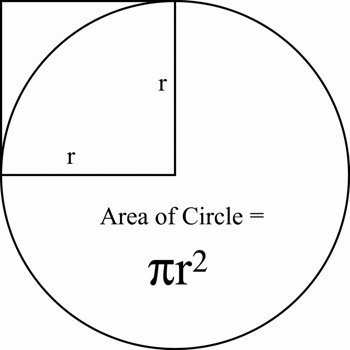Finding the Area of a Circle

The area of a circle can be found by multiplying pi (π = 3.14) by the square of the radius

If a circle has a radius of 4, its area is (3.14 * 4 * 4) = 50.24

If you know the diameter, the radius is 1/2 as large.The area of a circle is the number of square units inside that circle. If each square in the circle to the left has an area of 1 cm², you could count the total number of squares to get the area of this circle. Thus, if there were a total of 28.26 squares, the area of this circle would be 28.26 cm² However, it is easier to use one of the following formulas:

There is only one formula for the area of a circle: A = π r2

Example: The radius of a circle is 3 inches. What is the area?

Solution: Area = 3.14 x (3 in) x (3 in)

Area = 3.14 x (9 in)

Area = 28.26 in